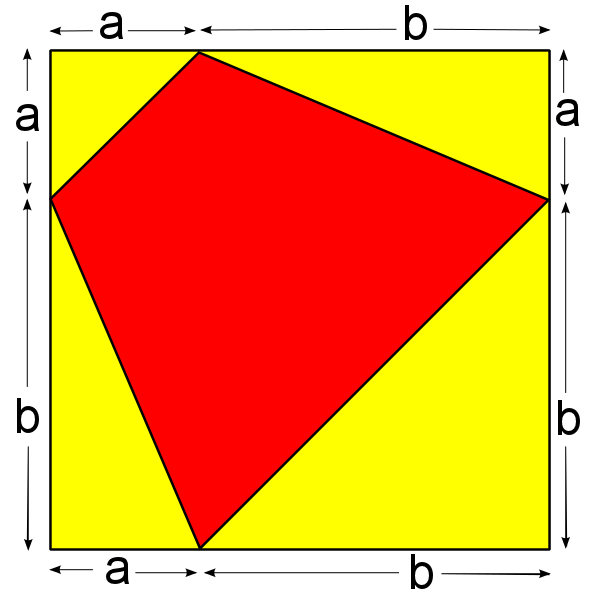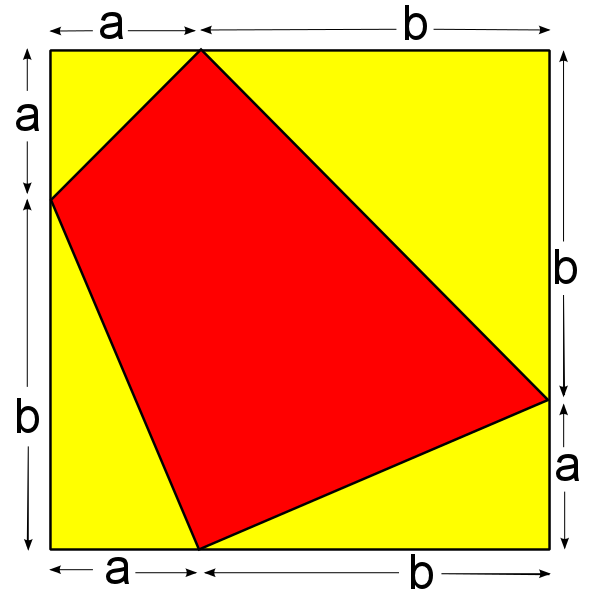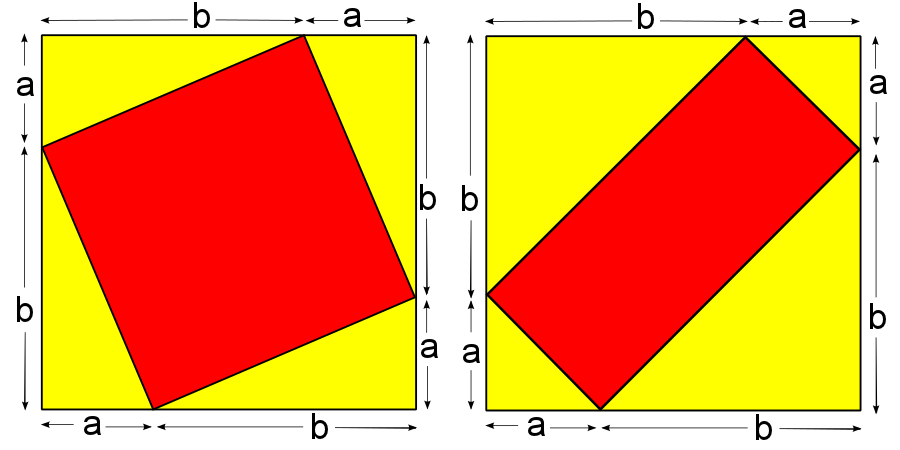#### You may also like### Kissing Triangles

Determine the total shaded area of the 'kissing triangles'.### Isosceles

Prove that a triangle with sides of length 5, 5 and 6 has the same area as a triangle with sides of length 5, 5 and 8. Find other pairs of non-congruent isosceles triangles which have equal areas.### Linkage

Four rods, two of length a and two of length b, are linked to form a kite. The linkage is moveable so that the angles change. What is the maximum area of the kite?

# Quadrilaterals in a Square

### Why do this problem?

This problem gives students the opportunity to prove a relationship in two different ways; algebraically, and geometrically. It follows on nicely from Triangles in a Square.

### Possible approach

This printable worksheet may be useful: Quadrilaterals in a Square.
The diagrams from the problem are available as slides.

Ask students to choose two different numbers between 1 and 8, and to construct the diagram below on squared paper using their two numbers a and b:"Can you work out the area of the quadrilateral formed in the square?"
Once students have had a chance to work out the area, collect on the board in two columns the area of the quadrilateral and the area of the square.

"What do you notice?" The quadrilateral has half the area of the square.
"Can we prove that it's true for every quadrilateral formed in this way?"

Students could work in pairs to try to come up with a proof. Encourage them to look for an algebraic and a geometric proof. The Solution has some examples of proofs.

Bring the class together and invite a couple of students to present their proofs. Then provide the second diagram:"Can you prove a similar result for this quadrilateral?"

Finally, students could work on the last part of the problem, showing that the two red areas sum to the area of the yellow square in the diagram below:### Key questions

Can you express each area algebraically?
Are there any lines you could draw?
Are there any shapes you could move in order to compare areas?

### Possible extension

Trapezium Four invites students to consider areas of triangles in a trapezium.

### Possible support

Triangles in a Square is a simpler problem where students might use similar strategies to find areas.